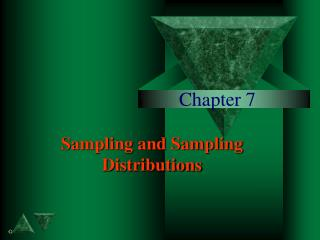DownloadDownload PresentationChapter 7

Chapter 7

Download PresentationChapter 7

- - - - - - - - - - - - - - - - - - - - - - - - - - - E N D - - - - - - - - - - - - - - - - - - - - - - - - - - -
Presentation Transcript

1. Chapter 7 Sampling and Sampling Distributions

2. Simple Random Sample Suppose that we want to select a sample of n objects from a population of N objects. A simple random sample is selected such that every object has an equal probability of being selected and the objects are selected independently - -the selection of one object does not change the probability of selecting any other objects.

3. Simple Random Sample Simple random samples are the ideal sample. In a number of real world sampling studies analysts develop alternative sampling procedures to lower the costs of sampling. But the basis for determining if these strategies are acceptable is to determine how closely they approximate a simple random sample.

4. Sampling Distributions Consider a random sample selected from a population to make an inference about some population characteristic, such as the population mean, by using a sample statistic such as a sample mean, X. The inference is based on the realization that every random sample would have a different number for X and thus X is a random variable. The sampling distribution of this statistic is the probability distribution of the values it could take over all possible samples of the same number of observations drawn from the population.

5. Sampling Distributions Consider a random sample selected from a population to make an inference about some population characteristic, such as the population mean, by using a sample statistic such as a sample mean, X. The inference is based on the realization that every random sample would have a different number for X and thus X is a random variable. The sampling distribution of this statistic is the probability distribution of the values it could take over all possible samples of the same number of observations drawn from the population.

6. Sample Mean Let X1, X2, . . . Xn be a random sample from a population. The sample mean value of these observations is defined as

7. Results for the Sampling Distribution of the Sample Mean Let X denote the sample mean of a random sample of n observations from a population with a mean X and variance 2. Then • The sampling distribution of X has mean • The sampling distribution of X has standard deviation This is called the standard error of X.

8. Results for the Sampling Distribution of the Sample Mean • If the sample size is not small compared to the population size N, then the standard error of X is • If the population distribution is normal, then the random variable Has a standard normal distribution with mean 0 and variance 1.

9. Standard Normal Distribution for the Sample Mean Whenever the sampling distribution of the sample mean is a normal distribution we can compute a standardized normal random variable, Z, that has mean 0 and variance 1

10. Central Limit Theorem Let X1, X2, . . . , Xn be a set of n independent random variables having identical distributions with mean  and variance 2, with X as the sum and X as the mean of these random variables. As n becomes large, the central limit theorem states that the distribution of approaches the standard normal distribution.

11. Sample Proportions Let Xbe the number of successes in a binomial sample of n observations, with parameter . The parameter  is the proportion of the population members that have a characteristic of interest. We define the sample proportion as The sum X is the sum of a set of n independent Bernoulli random variables each with a probability of success . As a result p is the mean of a set of independent random variables and the results developed in the previous sections for sample means apply. In addition the central limit theorem can be used to argue that the probability distribution for p can be modeled as a normal.

12. Sampling Distribution of the Sample Proportion Let p denote the sample proportion of successes in a random sample from a population with proportion of success . Then • The sampling distribution of p has mean  • The sampling distribution of p has standard deviation • If the sample size is large, the random variable is approximately distributed as a standard normal. The approximation is good if

13. Sample Variance Let X1, X2, . . . , Xn be a random sample from a population. The quantity Is called the sample variance and its square root s is called the sample standard deviation. Given a specific random sample we would compute the sample variance and the sample variance would be different for each random sample, because of differences in sample observations.

14. Sampling Distribution of the Sample Variances Let s2X denote the sample variance for a random sample of n observations from a population with variance 2. Then • The sampling distribution of s2 has mean 2 • The variance of the sampling distribution of s2X depends on the underlying population distribution. If that distribution is normal, then • If the population distribution is normal then (n-1)s2/ 2 is distributed as 2(n-1)